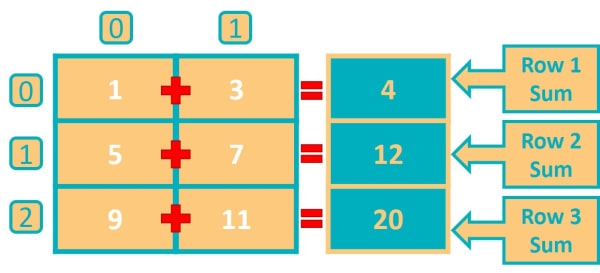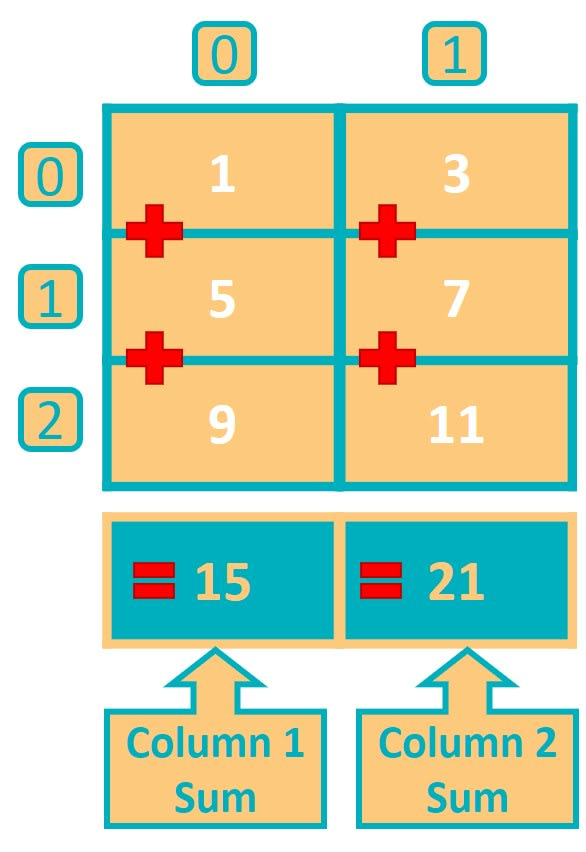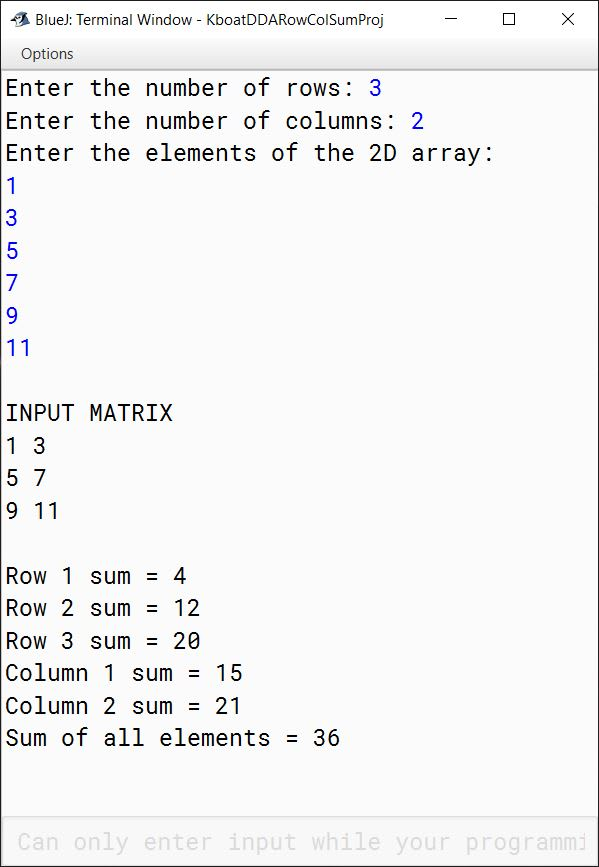# Finding Row-Wise Sum, Column-Wise Sum and Sum of All Elements of Double Dimensional Array

## ICSE Computer Applications

In this lesson we will learn about three things:

1. Computing the sum of all elements of the double dimensional array.
2. Computing the row-wise sum of the double dimensional array.
3. Computing the column-wise sum of the double dimensional array.

Let’s again take our 3 x 2 double dimensional array arr which we used in the previous lessons.

`int arr[][] = {  {1, 3}, {5, 7}, {9, 11} };`

Sum of all the elements of the array is straight-forward. You add up all the elements of the array and you will get the sum of all the elements.

`1 + 3 + 5 + 7 + 9 + 11 = 36`

Row-wise sum of the array means computing the sum of each row of the array.Adding 1 and 3 gives the sum of first row as 4. Adding 5 and 7 gives the sum of second row as 12. Adding 9 and 11 gives the sum of third row as 20.

For column-wise sum, we compute the sum of each column of the array.Summing up the first column gives 15 and second column gives 21.

## BlueJ Program to Calculate Row-wise Sum, Column-Wise Sum & Sum of All Elements of 2D Array

```import java.util.Scanner;

public class KboatDDARowColSum
{
public static void main(String args[]) {
Scanner in = new Scanner(System.in);

//Get the dimensions and array elements from the user
System.out.print("Enter the number of rows: ");
int rows = in.nextInt();
System.out.print("Enter the number of columns: ");
int cols = in.nextInt();
int arr[][] = new int[rows][cols];

System.out.println("Enter the elements of the 2D array:");
for (int i = 0; i < rows; i++) {
for (int j = 0; j < cols; j++) {
arr[i][j] = in.nextInt();
}
}

//Display the array in Matrix form
System.out.println();
System.out.println("INPUT MATRIX");

for (int i = 0; i < rows; i++) {
for (int j = 0; j < cols; j++) {
System.out.print(arr[i][j] + " ");
}
System.out.println();
}
System.out.println();

//Compute row-wise and sum of all elements
int sum = 0;
for (int i = 0; i < rows; i++) {
int rSum = 0;
for (int j = 0; j < cols; j++) {
sum += arr[i][j];
rSum += arr[i][j];
}
System.out.println("Row " + (i + 1) + " sum = " + rSum);
}

//Compute column-wise sum
for (int i = 0; i < cols; i++) {
int cSum = 0;
for (int j = 0; j < rows; j++) {
cSum += arr[j][i];
}
System.out.println("Column " + (i + 1) + " sum = " + cSum);
}

System.out.println("Sum of all elements = " + sum);
}
}```

#### OutputIn the above program, we first accept the array details from the user. After that, we display the array in matrix form. Then we compute the row-wise sum and sum of all elements. Finally, we compute the column-wise sum.

I have added the code to print the array in matrix form so that by looking at the output we can easily cross check if the sum values are computed correctly or not. If you are writing this program for your exams, then you can skip this part if the question does not explicitly mention about displaying the array in matrix form.

Coming to the part where we compute the row-wise sum and sum of all elements:

```//Compute row-wise and sum of all elements
int sum = 0;
for (int i = 0; i < rows; i++) {
int rSum = 0;
for (int j = 0; j < cols; j++) {
sum += arr[i][j];
rSum += arr[i][j];
}
System.out.println("Row " + (i + 1) + " sum = " + rSum);
}```

First, we declare an int variable `sum` and initialize it to zero. `sum` will hold the sum of all elements of the array. Next, we have the outer loop for the rows of the array and inner loop for the columns. With the help of these two loops, we traverse the array row-wise and add each element of the array to the int variable `sum`. To compute the sum of each row, we declare another int variable `rSum` just before the inner loop starts and initialize it to zero. Inside the inner loop we add the elements of the row to `rSum`. Once the inner loop completes executing, we have sum of the row in `rSum`. We then print it outside the inner loop. As `rSum` is declared inside the outer loop, it is initialized to zero with each iteration of the outer loop. This way, each iteration of outer loop computes and prints the sum of the corresponding row of the double dimensional array `arr`.

Next, let's look at the code to compute column-wise sum:

```//Compute column-wise sum
for (int i = 0; i < cols; i++) {
int cSum = 0;
for (int j = 0; j < rows; j++) {
cSum += arr[j][i];
}
System.out.println("Column " + (i + 1) + " sum = " + cSum);
}```

Column-Wise sum is like row-wise sum, just that now we need to traverse the double dimensional array column-wise rather than row-wise. To do this, we will again use two loops, but the outer loop will run for the number of columns and inner loop will run for the number of rows. Note that this is opposite to row-wise sum, there outer loop was running for number of rows and inner loop was running for number of columns. Now we need to go over the array column-wise so outer loop will run for number of columns and inner loop will run for number of rows.

The int variable `cSum` is like the `rSum` variable we saw earlier. It will accumulate the sum of each column. In the inner loop, we go over each element of the corresponding column and add it to `cSum`. Note that, in the line `cSum += arr[j][i];` the first subscript of arr is j and second is i unlike row-wise sum where i was first and j was second. Once the inner loop completes executing, we have sum of the column in `cSum`. We then print it outside the inner loop. Each iteration of outer loop computes and prints the sum of the corresponding column of the double dimensional array `arr`.